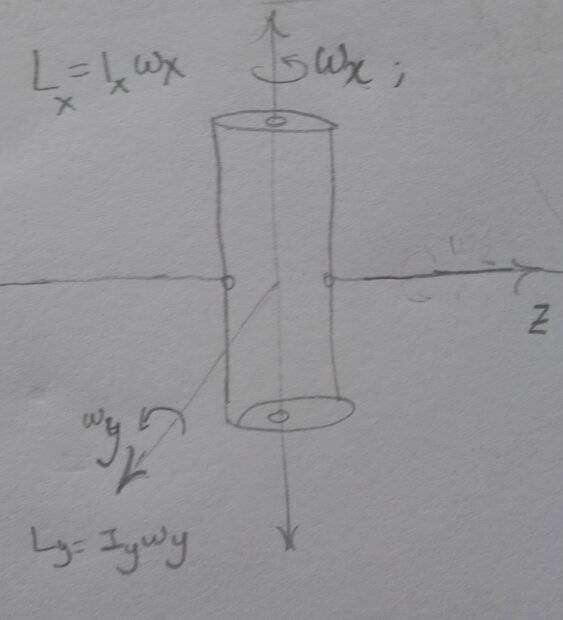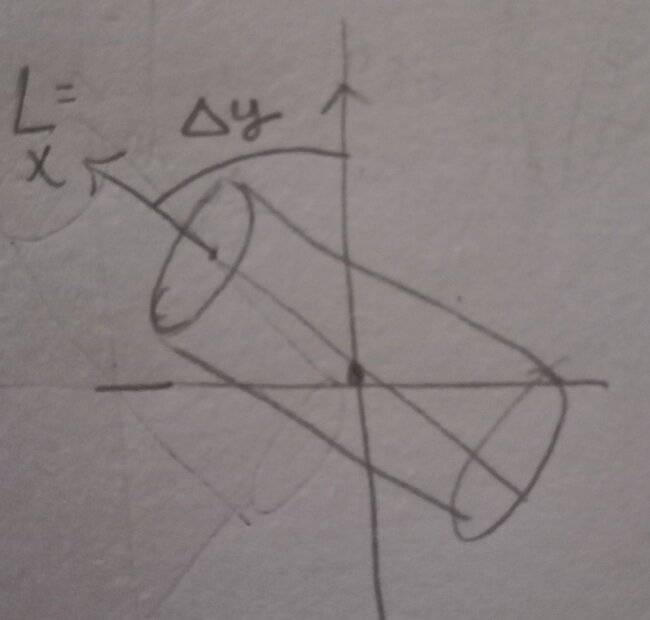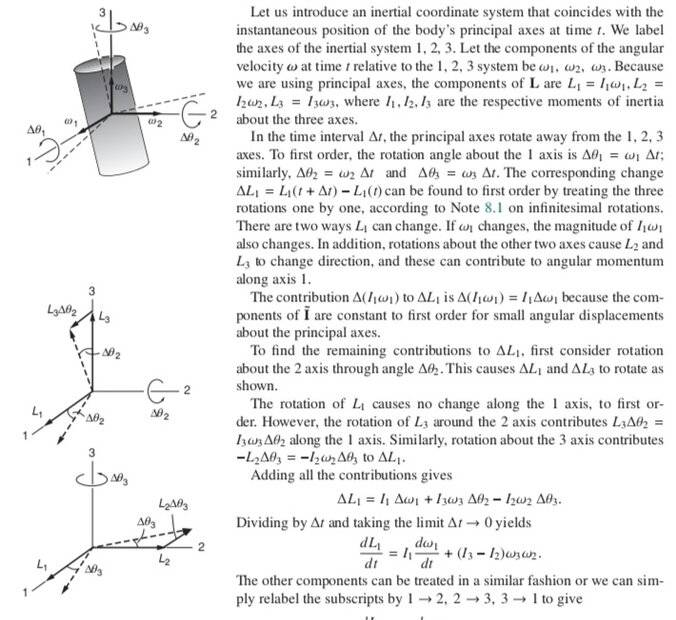# Rotation about two axes and angular momentum

• I
• Kashmir

#### KashmirI've a body having initial angular velocity at ## t=0 ## as shown. The axis shown are fixed in inertial space and initially match with the principal axis. I want to find the infinitesimal change at ##t+\Delta t## in the angular momentum along the ##z## axis.

I've seen the following approach which I don't understand:
One contribution to change in ##L_z## is due to rotation about y axis. This causes ##L_x## to rotate and hence a component ##-L_x \Delta{_y}## appears.How do we know that ##Lx## will remain constant in magnitude? Also the actual motion won't be as is shown, in which the body simply goes around the y-axis while maintaining it's spin ##L_x##

A similar method is used here by Kleppner and Kolenkow hereLast edited:
•Delta2
May we expect Lz=0? At least periodic it seems.

anuttarasammyak said:
May we expect Lz=0? At least periodic it seems.
Initially?

Yes, and I assume ##\mathbf{L}=(L_x,L_y,0)## is conserved in later time.

## 1. What is rotation about two axes?

Rotation about two axes refers to the motion of an object around two different axes simultaneously. This type of rotation is also known as compound rotation.

## 2. How is angular momentum defined?

Angular momentum is a measure of the rotational motion of an object and is defined as the product of its moment of inertia and its angular velocity.

## 3. How is angular momentum conserved in a system?

Angular momentum is conserved in a system when there are no external torques acting on the system. This means that the total angular momentum before and after an event or interaction remains the same.

## 4. Can angular momentum be negative?

Yes, angular momentum can be negative. The direction of angular momentum is determined by the direction of the angular velocity and the moment of inertia. If the angular velocity and moment of inertia are in opposite directions, the angular momentum will be negative.

## 5. How does rotation about two axes affect the stability of an object?

Rotation about two axes can affect the stability of an object by changing its moment of inertia. Objects with a larger moment of inertia tend to be more stable and resist changes in their rotation, while objects with a smaller moment of inertia can be more easily rotated or tipped over.